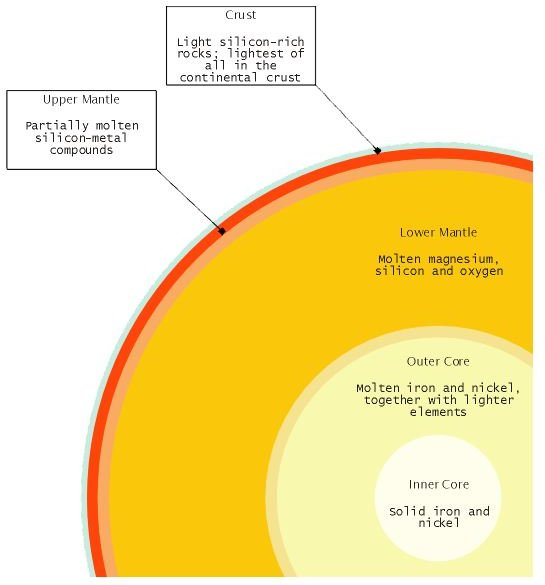# Learning the Meaning of Density in Earth Science

## What is Density?

Density is a physical property that measures the mass of a given material per volume unit. It can be calculated by a simple formula that divides the mass by the volume of a body:

ρ = m/V, where ρ is the density, m the mass and V the volume of the body.

The unit for measuring density is the kilogram per cubic meter or kg/m3 in the SI system. Another unit that is frequently used, is the gram per cubic centimeter or g/cm3. In the British Imperial System, we use pounds per cubic foot (lb/cu ft), ounces per cubic inch (oz/cu in) and a number of other density units.

The formula calculation is quite straightforward, as long as the mass and the volume are known. But what is the meaning of density? It is actually a measure indicating how densely the molecules of a material or substance are packed together. This formula is accurate when the body is homogenous. In the case that a body is heterogenous, we calculate an average value, the "mean density".

## The ProblemThe difficulty in calculating an accurate value for the Earth's density lies in its heterogeneity. More specifically, our planet consists of three main parts, the crust (continental and oceanic), the mantle, and the core (outer and inner), all of them having different density values:

Continental Crust: 2.7 – 3.0 g/cm3

Oceanic Crust: 3.0 – 3.3 g/cm3

Mantle: 3.3 – 5.7 g/cm3

Outer Core: 9.9 – 12.2 g/cm3

Inner Core: 12.6 – 13.0 g/cm3

For further information on these terms, check the article: "Earth's Structure Vocabulary Terms"

These mean density values are being constantly corrected/calculated to be more accurate by earthquake analysis and laboratory experiments on rock samples. Taking into account the relative thickness of each layer, we can calculate the respective percentages of each layer's contribution to the mean density.

## Calculations

The first scientist that calculated the mass and mean density of the Earth was Henry Cavendish. He also determined Newton's gravitational constant through the well-known Cavendish Experiment. The experiment is based on a hand-made device where two lead balls are attached to a suspended metal rod. By placing metal masses near the hanging balls, he managed to calculate the force of attraction between them and subsequently, the density of these masses. He then applied the same method for measuring Earth's attraction force and density.

According to his results (and to modern corrections), the mean density of our planet is in the range of 5.5 to 5.52 g/cm3. This is the range of values found in any bibliographic source. As far as the volume and the mass are concerned, things are a little more complicated. Earth is not a perfect sphere, so the formula used for calculating the volume of a sphere is not completely accurate: 4/3πr3, where π is the mathematical constant equal to 3.14159 and r the radius of the Earth. Earth's diameter at the equator is 12756.75 km and therefore the radius is exactly half or 6378.375 km. After taking into account the most advanced methods of calculation, the following values are generally accepted:

Earth mean density: 5.515 g/cm3

Earth Mass: 5.9736×1024 kg

Earth Volume: 1.08321x 1012 km3

## The Rest of the Solar System

The following list includes the mean density values of the Sun and the planets of our Solar System:

Sun: 14.08 g/cm3

Mercury: 5.43 g/cm3

Venus: 5.243 g/cm3

Mars: 3.934 g/cm3

Jupiter: 1.326 g/cm3

Saturn: 0.687 g/cm3

Uranus: 1.270 g/cm3

Neptune: 1.638 g/cm3

If we accept a value of 5.52 g/cm3, we can see that Earth's density is higher than that of any other planet, meaning that it is the densest planet in the Solar System. The reason for this fact, lies in the fact that there are no major gaseous components like the ones in the gas giants. On the contrary, all its mass is concentrated into heavy solids and therefore, despite its small volume, it still has the highest mean value among the rest of the planets. Finally, Earth's large mass results in greater gravitational forces. These forces affect its shape and density by compressing its layers to a much larger degree compared to the other inner planets.

To make some further comparisons: the density of the Moon is 3.346 g/cm3 and Jupiter's Io is 3.53 g/cm3. Io is actually the densest moon of our Solar System, while our Moon comes in second place. Similarly to Earth, the Moon has two layers: a 65-kilometer crust and an inner mantle reaching a 3,700 kilometer depth. This second layer however is much denser than the crust.